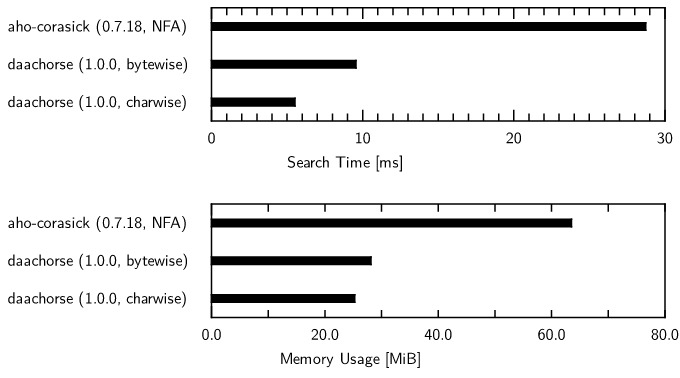## no-std daachorse

Daachorse: Double-Array Aho-Corasick

### 11 releases(1 stable)

 1.0.0 Aug 1, 2022 Jul 7, 2022 Jun 7, 2022 Mar 6, 2022 Nov 1, 2021

#39 in Text processing

Used in 6 crates (4 directly)

MIT/Apache

165KB
3K SLoC

# 🐎 daachorse: Double-Array Aho-Corasick

A fast implementation of the Aho-Corasick algorithm using the compact double-array data structure.

The main technical ideas behind this library appear in this paper.

## Overview

Daachorse is a crate for fast multiple pattern matching using the Aho-Corasick algorithm, running in linear time over the length of the input text. This crate uses the compact double-array data structure for implementing the pattern match automaton for time and memory efficiency. The data structure not only supports constant-time state-to-state traversal but also represents each state in the space of only 12 bytes.

For example, compared to the NFA of the aho-corasick crate, which is the most popular Aho-Corasick implementation in Rust, Daachorse can perform pattern matching 3.0–5.2 times faster while consuming 56–60% smaller memory when using a word dictionary of 675K patterns. Other experimental results are available on Wiki.## Requirements

Rust 1.58 or higher is required to build this crate.

## Example usage

Daachorse contains some search options, ranging from standard matching with the Aho-Corasick algorithm to trickier matching. They will run very fast based on the double-array data structure and can be easily plugged into your application, as shown below.

### Finding overlapped occurrences

To search for all occurrences of registered patterns that allow for positional overlap in the input text, use `find_overlapping_iter()`. When you use `new()` for construction, the library assigns a unique identifier to each pattern in the input order. The match result has the byte positions of the occurrence and its identifier.

``````use daachorse::DoubleArrayAhoCorasick;

let patterns = vec!["bcd", "ab", "a"];
let pma = DoubleArrayAhoCorasick::new(patterns).unwrap();

let mut it = pma.find_overlapping_iter("abcd");

let m = it.next().unwrap();
assert_eq!((0, 1, 2), (m.start(), m.end(), m.value()));

let m = it.next().unwrap();
assert_eq!((0, 2, 1), (m.start(), m.end(), m.value()));

let m = it.next().unwrap();
assert_eq!((1, 4, 0), (m.start(), m.end(), m.value()));

assert_eq!(None, it.next());
``````

### Finding non-overlapped occurrences with the standard matching

If you do not want to allow positional overlap, use `find_iter()` instead. It performs the search on the Aho-Corasick automaton and reports patterns first found in each iteration.

``````use daachorse::DoubleArrayAhoCorasick;

let patterns = vec!["bcd", "ab", "a"];
let pma = DoubleArrayAhoCorasick::new(patterns).unwrap();

let mut it = pma.find_iter("abcd");

let m = it.next().unwrap();
assert_eq!((0, 1, 2), (m.start(), m.end(), m.value()));

let m = it.next().unwrap();
assert_eq!((1, 4, 0), (m.start(), m.end(), m.value()));

assert_eq!(None, it.next());
``````

### Finding non-overlapped occurrences with the longest matching

If you want to search for the longest pattern without positional overlap in each iteration, use `leftmost_find_iter()` with specifying `MatchKind::LeftmostLongest` in the construction.

``````use daachorse::{DoubleArrayAhoCorasickBuilder, MatchKind};

let patterns = vec!["ab", "a", "abcd"];
let pma = DoubleArrayAhoCorasickBuilder::new()
.match_kind(MatchKind::LeftmostLongest)
.build(&patterns)
.unwrap();

let mut it = pma.leftmost_find_iter("abcd");

let m = it.next().unwrap();
assert_eq!((0, 4, 2), (m.start(), m.end(), m.value()));

assert_eq!(None, it.next());
``````

### Finding non-overlapped occurrences with the leftmost-first matching

If you want to find the earliest registered pattern among ones starting from the search position, use `leftmost_find_iter()` with specifying `MatchKind::LeftmostFirst`.

This is the so-called leftmost first match, a tricky search option supported in the aho-corasick crate. For example, in the following code, `ab` is reported because it is the earliest registered one.

``````use daachorse::{DoubleArrayAhoCorasickBuilder, MatchKind};

let patterns = vec!["ab", "a", "abcd"];
let pma = DoubleArrayAhoCorasickBuilder::new()
.match_kind(MatchKind::LeftmostFirst)
.build(&patterns)
.unwrap();

let mut it = pma.leftmost_find_iter("abcd");

let m = it.next().unwrap();
assert_eq!((0, 2, 0), (m.start(), m.end(), m.value()));

assert_eq!(None, it.next());
``````

### Associating arbitrary values with patterns

To build the automaton from pairs of a pattern and user-defined value, instead of assigning identifiers automatically, use `with_values()`.

``````use daachorse::DoubleArrayAhoCorasick;

let patvals = vec![("bcd", 0), ("ab", 10), ("a", 20)];
let pma = DoubleArrayAhoCorasick::with_values(patvals).unwrap();

let mut it = pma.find_overlapping_iter("abcd");

let m = it.next().unwrap();
assert_eq!((0, 1, 20), (m.start(), m.end(), m.value()));

let m = it.next().unwrap();
assert_eq!((0, 2, 10), (m.start(), m.end(), m.value()));

let m = it.next().unwrap();
assert_eq!((1, 4, 0), (m.start(), m.end(), m.value()));

assert_eq!(None, it.next());
``````

### Building faster automata on multibyte characters

To build a faster automaton on multibyte characters, use `CharwiseDoubleArrayAhoCorasick` instead.

The standard version `DoubleArrayAhoCorasick` handles strings as UTF-8 sequences and defines transition labels using byte values. On the other hand, `CharwiseDoubleArrayAhoCorasick` uses Unicode code point values, reducing the number of transitions and faster matching.

``````use daachorse::CharwiseDoubleArrayAhoCorasick;

let patterns = vec!["全世界", "世界", "に"];
let pma = CharwiseDoubleArrayAhoCorasick::new(patterns).unwrap();

let mut it = pma.find_iter("全世界中に");

let m = it.next().unwrap();
assert_eq!((0, 9, 0), (m.start(), m.end(), m.value()));

let m = it.next().unwrap();
assert_eq!((12, 15, 2), (m.start(), m.end(), m.value()));

assert_eq!(None, it.next());
``````

## `no_std`

Daachorse has no dependency on `std` (but requires a global allocator with the `alloc` crate).

## CLI

This repository contains a command-line interface named `daacfind` for searching patterns in text files.

``````% cat ./pat.txt
fn
const fn
pub fn
unsafe fn
% find . -name "*.rs" | xargs cargo run --release -p daacfind -- --color=auto -nf ./pat.txt
...
...
./src/errors.rs:67:    fn fmt(&self, f: &mut fmt::Formatter) -> fmt::Result {
./src/errors.rs:81:    fn fmt(&self, f: &mut fmt::Formatter) -> fmt::Result {
./src/lib.rs:115:    fn default() -> Self {
./src/lib.rs:126:    pub fn base(&self) -> Option<u32> {
./src/lib.rs:131:    pub const fn check(&self) -> u8 {
./src/lib.rs:136:    pub const fn fail(&self) -> u32 {
...
...
``````

For software under `bench/data`, follow the license terms of each software.